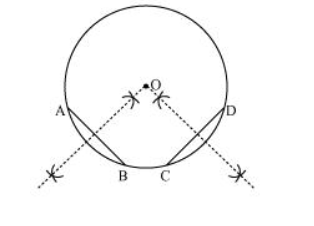# Suppose you are given a circle.

Question. Suppose you are given a circle. Give a construction to find its centre

Solution:

The below given steps will be followed to find the centre of the given circle.

Step1. Take the given circle.

Step2. Take any two different chords AB and CD of this circle and draw perpendicular bisectors of these chords.

Step3. Let these perpendicular bisectors meet at point O. Hence, O is the centre of the given circle.